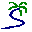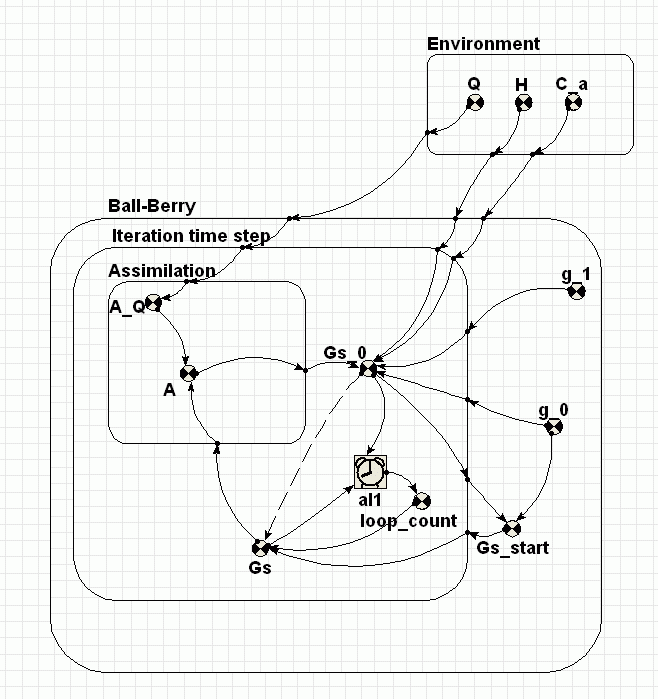# Model entry

Iterative solution to Ball-Berry stomatal conductance simultaneous equations (Simile V4+) - Model catalogue - Simulistics.com

 Search Simulistics Model catalogue Listed by keyword Listed by ID Listed by title Listed by date added

# Iterative solution to Ball-Berry stomatal conductance simultaneous equations (Simile V4+)

Model : BallBerry4a
Simile version : 4.0
Keywords : Stomatal conductance ; Evapotranspiration ; Ecophysiology ; Technique ; Iteration ;

## Description

The Ball-Berry stomatal conductance poses a problem for conventional System Dynamics modelling tools because it depends upon the solution of the following pair of simultaneous equations.

Gs = g0 + g1 * A * H / Ca
A = Gs * AQ

Apart from the external parameters, g0, g1, H, Ca and AQ, the equation for Gs depends only on A, and the equation for A depends only on Gs. In this implementation, the loop is opened, by introducing a place-holder for Gs, called Gs_0. This variable is calculated from A. A is calculated using Gs. A guess is made for the initial value of Gs. Subsequently, Gs is set to the last calculated value of Gs_0. The influence arrow from Gs_0 to Gs is dashed, indicating that the value from the previous iteration is to be used. The alarm symbol terminates the iterative loop when Gs and Gs_0 differ by less than one part in one thousand.

That�s all there is to it, except that it�s worth noting what to do if the iteration does not converge. It is necessary to use the new �Abort Execution� command in the �Model� menu of the model diagram window (i.e. NOT in the run control window).

## Files

### Model file

Click on the icon to download the model file. (You will need Simile to examine and run the model. A free evaluation version is available from the products page.)

Some browsers may attempt to display the model file, rather than open it in Simile; in this case, use the browser back button to return to this page, and use the context menu (invoked by right-clicking on the link) to save the target file to disk.BallBerry4aP.sml

## Diagram## Equations

```Equations in BallBerry4aP

Equations in Environment

Variable   C_a : Carbon dioxide concentration (umol CO2 (mol air)^-1)
C_a = graph(time())
Typical diurnal curve in forest canopy

Variable   H : Relative humidity (proportion)
H = graph(time())
Typical diurnal graph (24 hour)

Variable   Q : Photon flux density (umol m^-2 s^-1)
Q = graph(time())
Graph for a sunny day (24 hours)

Equations in Ball-Berry

Variable   Gs_start
Gs_start = if time()==0 then g_0 else last(Gs_0)
Where:
Gs_0=Iteration time step/Gs_0

Variable   g_0 : Stomatal conductance in the dark (mol m^-2 s^-1)
g_0 = 0.01

Variable   g_1 : Ball-Berry stomatal conductance coefficient
g_1 = 23

Equations in Iteration time step

Alarm
Variable   Gs
Gs = if loop_count==0 then Gs_start else Gs_0
Where:
Gs_start=../Gs_start

Variable   Gs_0 : Stomatal conductance (mol m^-2 s^-1)
Gs_0 = g_0+g_1*A*H/C_a
Where:
A=Assimilation/A
H=../../Environment/H
C_a=../../Environment/C_a
g_0=../g_0
g_1=../g_1
Ball-Berry equation

Variable   loop_count
loop_count = iterations(al1)

Equations in Assimilation

Variable   A : Assimation (umol CO2 m^-2 s^-1)
A = A_Q*Gs
Where:
Gs=../Gs

Variable   A_Q : Assimilation light response curve
A_Q = graph(Q)
Where:
Q=../../../Environment/Q
||